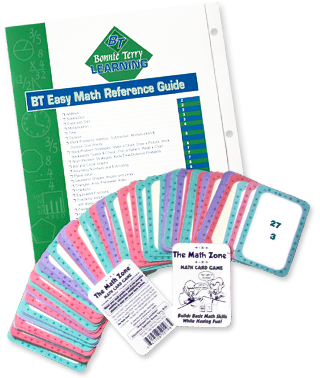# BT Easy Math Reference GuidePrice: \$42.00

## The Ultimate Guide for Math

The Bonnie Terry Math Pack consists of a game (The Math Zone), a guide (The BT Easy Math Reference Guide), and Super Spacers (20 paper organizers) that address the 3 roadblocks to math success, plus a FREE Bonus.

##### Your child will easily be able to:
• Add, subtract, multiply, and divide.
• Calculate fractions, decimals, and percents.
• Calculate area, perimeter, and ratio.
• Recognize and do calculations with geometric shapes, lines, and angle.
• Find clue words for word problems.
• Understand math vocabulary.

### A Memory and Math Concepts Game

A calculation practice game where children add, subtract, multiply, divide, halve the answer, or double their answers. Players learn how numbers grow and shrink with different mathematical calculations.

### The Ultimate Guide for Math

Gives children of all ages, from elementary school through college, the code, the secrets, and the tools they would need to solve all elementary math calculations right up to fractions, decimals, and percents.

### 2 Packs of 20 Paper Organizers

Gives children of all ages perfect spacing for math problems. Now they have neat and organized papers!

##### Bonus

A FREE Tip Sheet on getting the best use of the BT Easy Math Reference Guide.

Imagine how confident and proud your kids will feel about themselves when they are able to do their math homework on their own and get it right! Imagine your child actually enjoying math, even playing a math game. There will be a time that they even solve word problems with ease and enjoy doing them because they are interested in the story of the problem. That is why my daughter likes to do math problems – she likes the stories! Your child will be able to understand math vocabulary so solving the problems will be like child’s play. And their assignment will even be done neatly, where problems don’t run into each other. This can happen… this can be your child too!

#### The Math Pack

Price: \$35.00

##### Guarantee

As with all Bonnie Terry Learning Products, The Math Pack comes with a 30-day return.